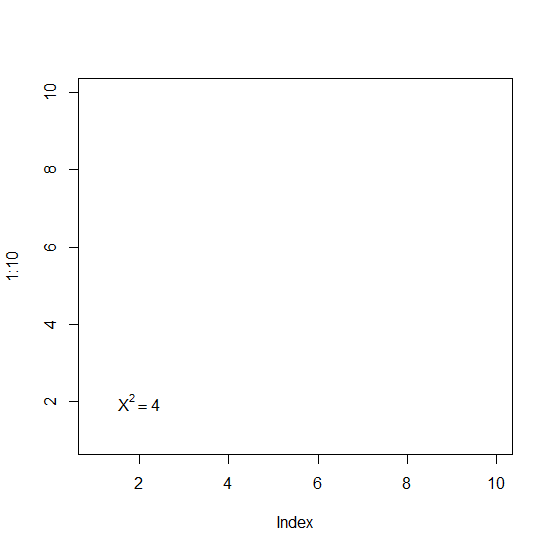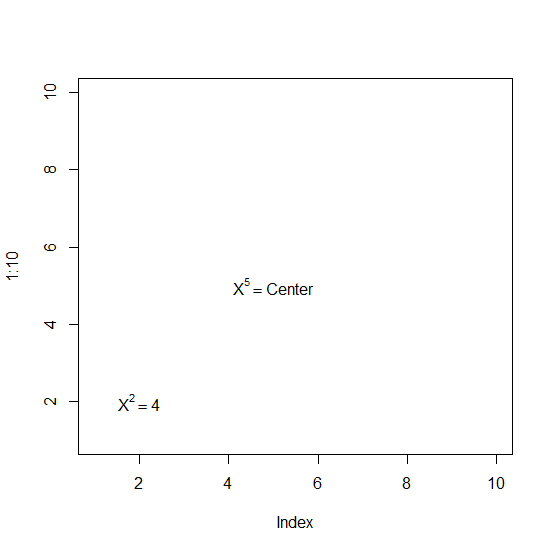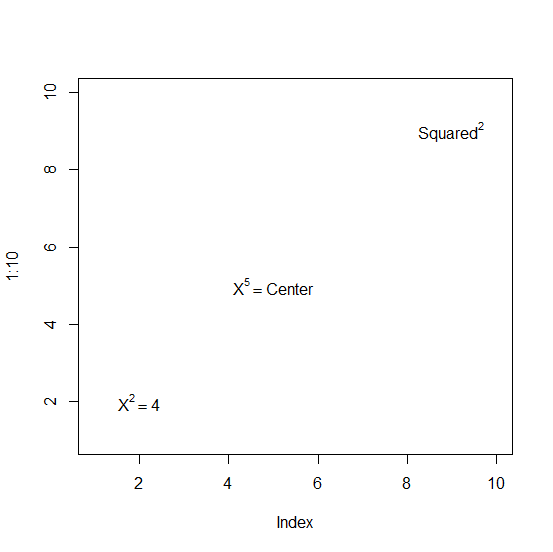# How to display the superscript for a single variable in a base R plot?

To display the superscript in a base R plot, we would need to use expression function inside text function. For example, if we want to display X-square inside a blank plot in base R then we can use the below code:

plot(1:10,type="n")
text(2,2,expression(X^2))

## Example1

> plot(1:10,type="n")
> text(2,2,expression(X^2==4))

## Output:## Example2

> text(5,5,expression(X^5==Center))

## Output:## Example3

> text(9,9,expression(Squared^2))

## Output: